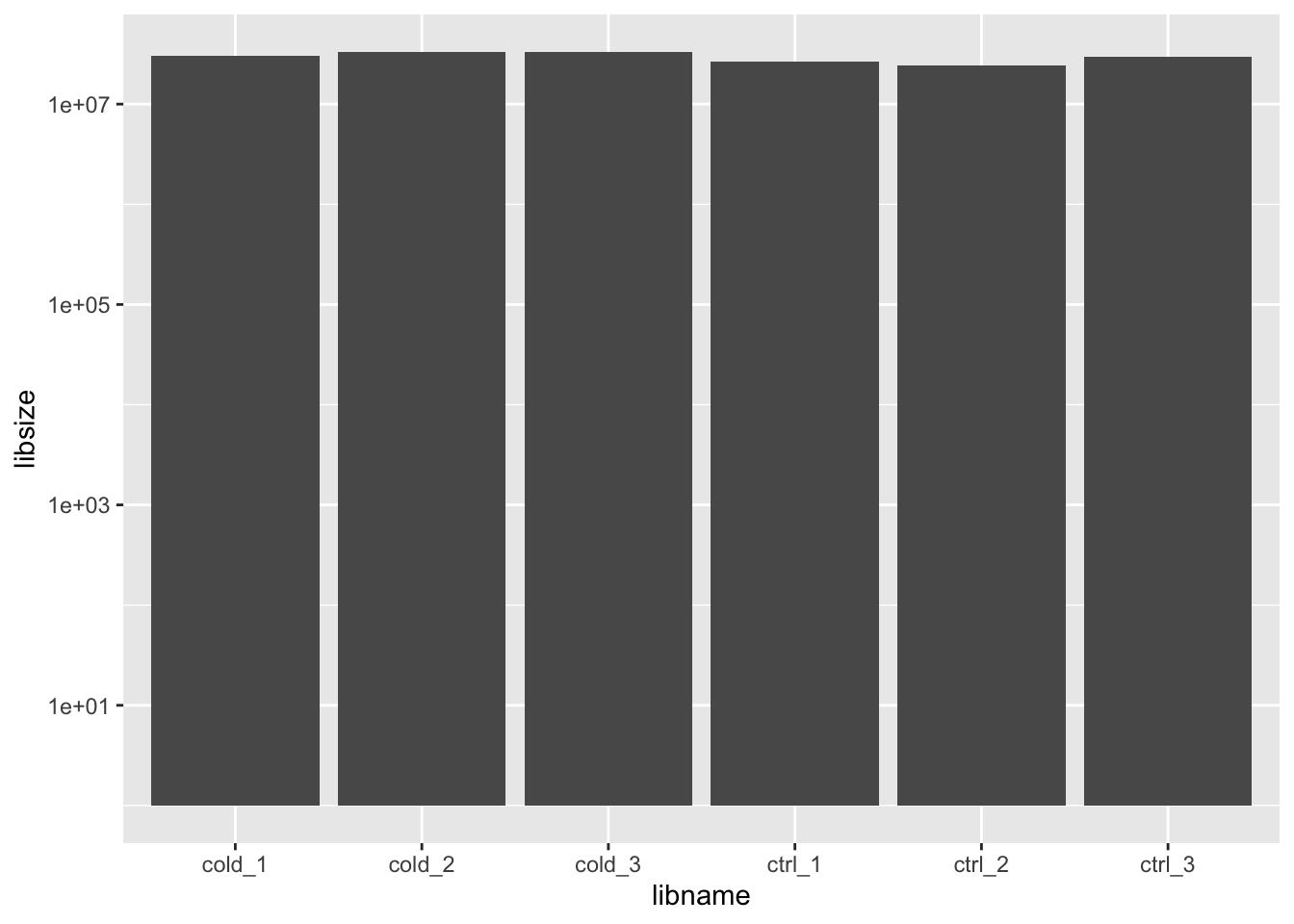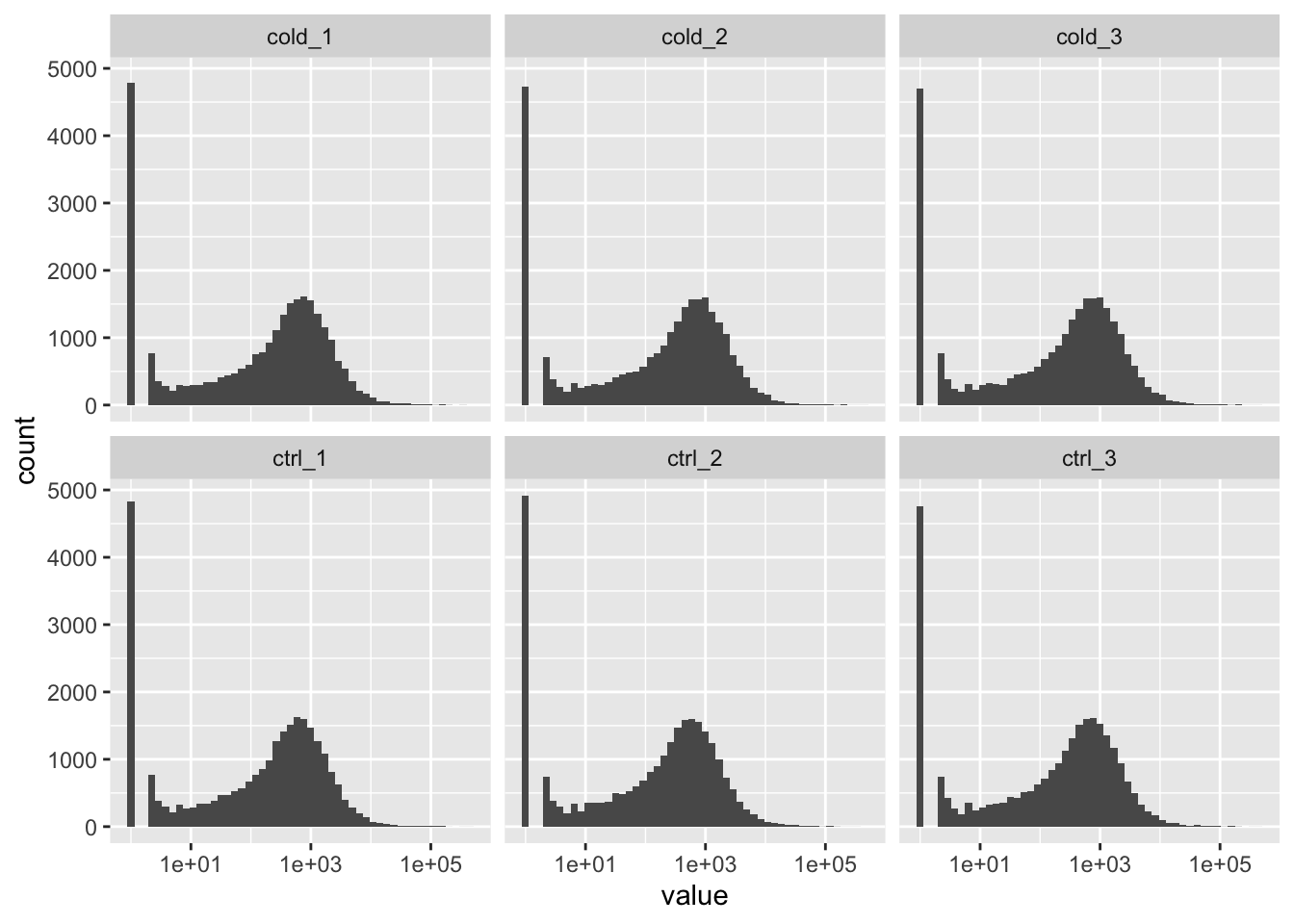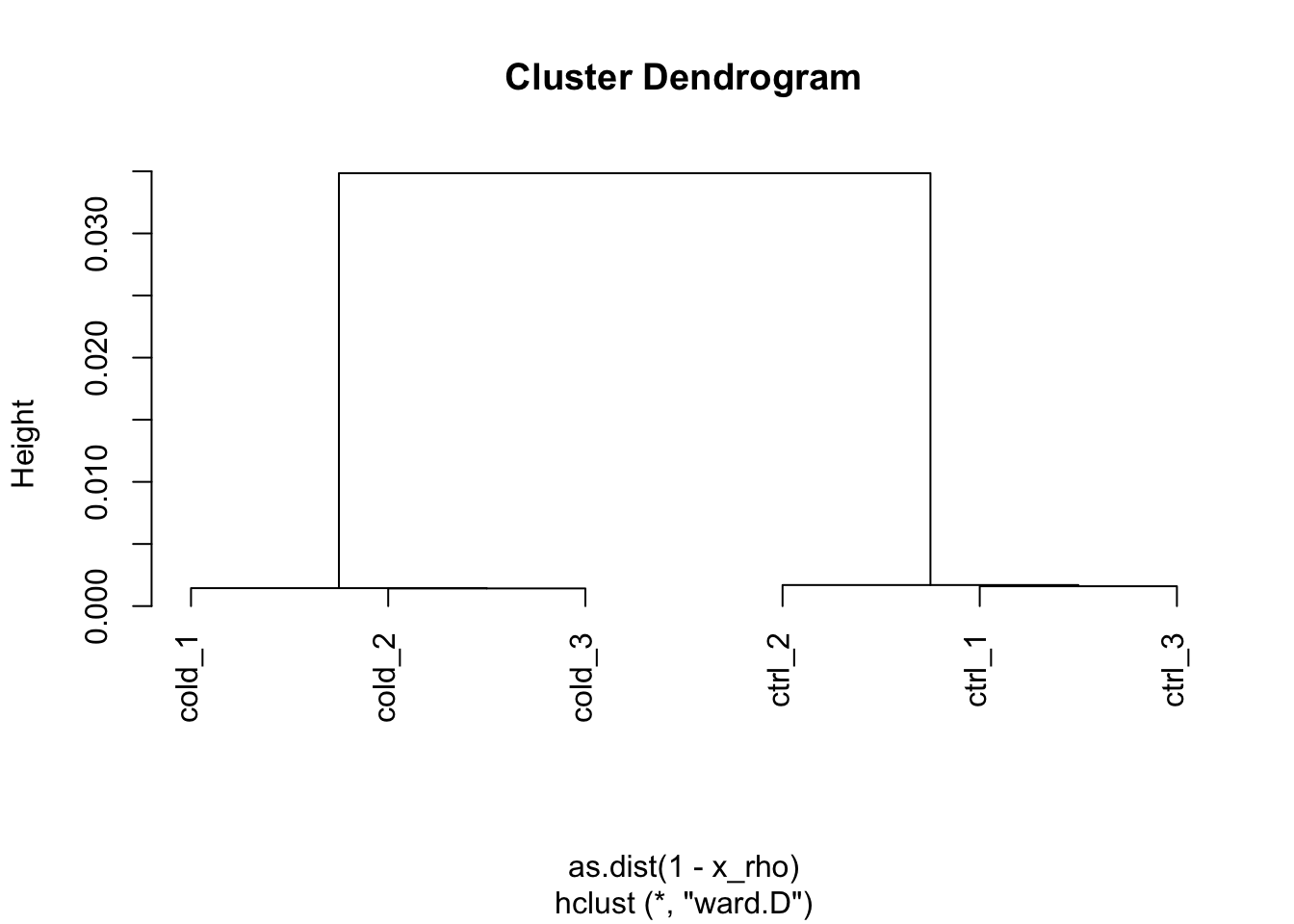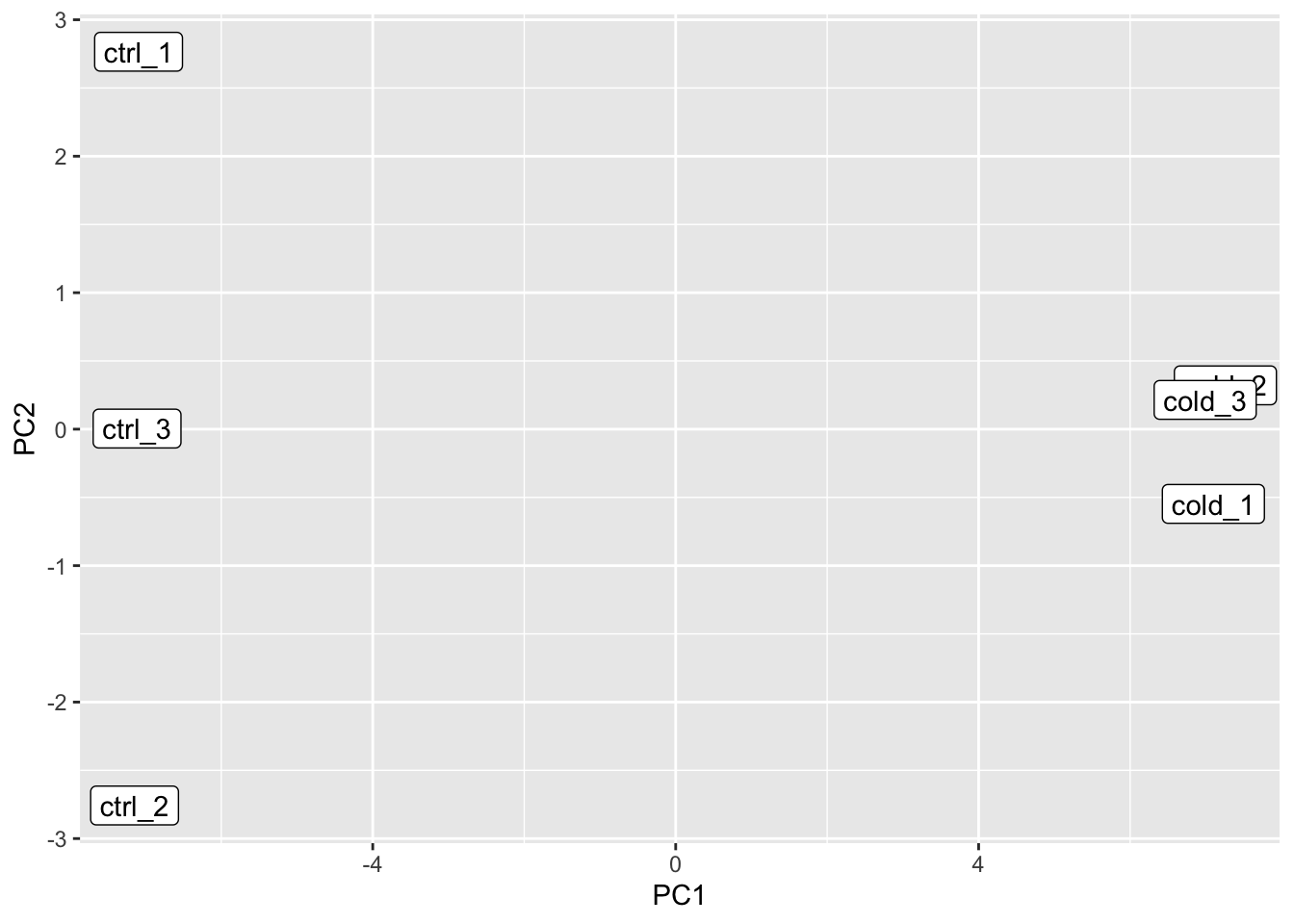This tutorial introduces a standard analysis precedures for differential expression (DE) analyses with RNA-Seq data. We aim to (i) identify differentially expressed genes (DEGs) from RNA-Seq data (also called as read count data), and (ii) unravel the biological functions of these DEGs. Since there are two types of DEG identification procedures, count-based and FPKM-based, we will introduces both procedures in this tutorial.

In this tutorial, we use a simply designed experimental dataset to study DE analyses. The dataset, obtained from Arabidopsis thaliana, consists of two groups, control and mutation groups. The control group consists of three biological replicates, and the mutation group also consists of three biological replicates. We will compare the two groups, and identify DEGs between the two groups.

# Analysis environment

RNA-Seq data analysis requires some specific packages, for example, edgeR and DESeq2 for DEG identification, clusterProfiler for gene ontology (GO) enrichment anlaysis. It does not like common R packages, these packages are usually reposited on Bioconductor. Here, we start R, and install the required packages from Bioconductor. In addition, for convenience, we also install some useful packages for data manipulation from CRAN.

``````# Bioconductor packages
if (!requireNamespace("BiocManager", quietly = TRUE))
install.packages("BiocManager")

BiocManager::install(c("edgeR", "DESeq2", "clusterProfiler", "org.At.tair.db", "ballgown", "genefilter"))

# CRAN packages
install.packages(c("tidyverse", "ggsci", "gplots"))``````

After the installation of R/Bioconductor packages, we restart R, and load the packages.

``````library(tidyverse)
library(ggsci)
library(gplots)
library(edgeR)              # DE analysis
library(DESeq2)             # DE analysis
library(ballgown)           # DE analysis
library(genefilter)         # DE analysis
library(clusterProfiler)    # GO analysis
library(DOSE)               # GO analysis
library(enrichplot)         # GO analysis
library(org.At.tair.db)     # GO analysis``````

To enable R find the count data, we set R working directory.

``setwd('~/projects/atrnaseq')``

# Count-based DEG identification

The count-based procedures use raw read counts directly for DEG identification. The procedures takes three steps to identify DEGs: (i) normalize counts (only adjust the library size), (ii) estimate dispersions, and (iii) perfrom statistical test (LRT, Wald test). There are two packages named edgeR and DESeq famouse for DEG identification.

## Overview

Overviews of whole datasets are essentkial steps of data anlaysis. In this section, we will summarise the statistics of this dataset and visualize them with charts, to check whether the data is suitable for analyses or not. First, we load expression data with `read.table` function.

``````d <- read.table('count/counts.txt', sep = '\t', header = TRUE)

Since most packages requires count data as a matrix type object, we format the loaded data into a matrix type, and set up row name and column name.

``````x <- as.matrix(d[, 7:ncol(d)])
rownames(x) <- sapply(strsplit(d[, 1], ':'), '[', 2)
colnames(x) <- c('ctrl_1', 'ctrl_2', 'ctrl_3', 'cold_1', 'cold_2', 'cold_3')
``````##           ctrl_1 ctrl_2 ctrl_3 cold_1 cold_2 cold_3
## AT1G01010    233    200    287    273    270    292
## AT1G01020    374    351    417    474    496    493
## AT1G01030     80     71     90     98    123    114
## AT1G01040   1729   1604   1962   1684   1866   1967
## AT1G01050   1946   1755   2083   2297   2451   2440
## AT1G01060    315    275    401    213    272    222``````

The total number of reads, library size, in a library reflects the sequencing performance. We use bar chart to visualize the library size. From the figure, we can see that the six libraries are similar to each other, and there is no outlier among these libraries.

``````# barplot(colSums(x))

fig_libsize <- data.frame(libname = colnames(x), libsize = colSums(x)) %>%
ggplot(aes(x = libname, y = libsize)) +
scale_y_log10() +
geom_bar(stat = 'identity')
print(fig_libsize)``````Next, we are going to check the distribution of read count of each library. We can see that each library has a sharp peak at zero of x-axis and a gentle peak between 1e2 and 1e3. The x-axis of two peaks among the six libraries are similar to each other.

``````# hist(log10(x[, 1]+ 1))

fig_countdist <- as.data.frame(x) %>%
pivot_longer(everything(), names_to = 'library') %>%
mutate(value = value + 1) %>%
ggplot(aes(x = value)) +
geom_histogram(bins = 50) +
scale_x_log10() +
facet_wrap(~ library, nrow = 2)
print(fig_countdist)``````Sample (library) clustering gives us insights for discussion and chances to explore outliers in the dataset. Hierarchical clustering and PCA are commonly used for this purpose. Hierarchical clustering can be anlayzed by calculating spearman correlation coefficients between two libraries across all combinations. To avoid sequencing errors, usually, we only use the highly expressed genes for clustering.

From the clustering result, we can see that there were two clusters: (i) one contains three replicates of control group, and (ii) the other contains three replicates of mutation group. The clustering result reflects the design of this experiments.

``````x_log10 <- log10(sweep(x, 2, 1e6 / colSums(x), '*') + 1)
x_log10_highexp <- x_log10[rowSums(x_log10 > 0) > 3, ]

# only use high expressed genes
x_rho <- cor(x_log10_highexp, method = 'spearman')
x_hist <- hclust(as.dist(1 - x_rho), method = 'ward.D')
plot(x_hist, h = -1)``````We then use highly expressed genes to perform PCA and plot the first and second principal components. We can see that replicates in the control group are extreamly different from replicates in the mutation group.

``````x_log10 <- log10(sweep(x, 2, 1e6 / colSums(x), '*') + 1)
x_log10_highexp <- x_log10[rowSums(x_log10 > 0) > 3, ]

# only use high expressed genes
x_pca <- prcomp(t(x_log10_highexp), scale = FALSE)

# plot(x_pca\$x[, c('PC1', 'PC2')])
# text(x_pca\$x[, c('PC1', 'PC2')], labels = rownames(x_pca\$x))

fig_pca <- as.data.frame(x_pca\$x) %>%
mutate(libname = rownames(x_pca\$x)) %>%
ggplot(aes(x = PC1, y = PC2, label = libname)) +
geom_label()
print(fig_pca)``````## DEG identification (edgeR)

Differential expression analysis aims to idenitfy the genes that are differentially expressed between two groups. To use edgeR for DEG identification, we prepare the group information and experimental design for the count data.

``````x_group <- factor(c(1, 1, 1, 2, 2, 2))
x_design <- model.matrix(~ x_group)
print(x_design)``````
``````##   (Intercept) x_group2
## 1           1        0
## 2           1        0
## 3           1        0
## 4           1        1
## 5           1        1
## 6           1        1
## attr(,"assign")
##  0 1
## attr(,"contrasts")
## attr(,"contrasts")\$x_group
##  "contr.treatment"``````

Then, we set up count data and group information to edgeR class object. Since genes with low expression affects accuracy of statistical test, we use `filterByExpr` function with default parameters to remove the low expressed genes.

``````y <- DGEList(counts = x, group = x_group)
keep <- filterByExpr(y)
y <- y[keep, , keep.lib.sizes = FALSE]``````

We use MDS plot to check the distance among the replicates. MDS reuslt should be similar to the reuslts of PCA and hierachical clustering.

``plotMDS(y)``